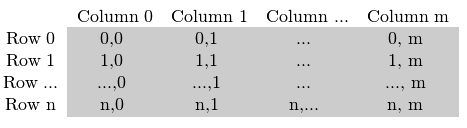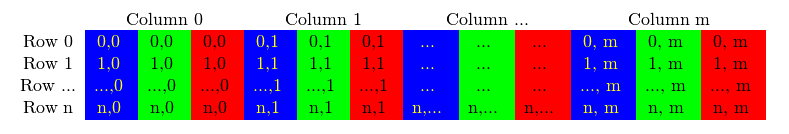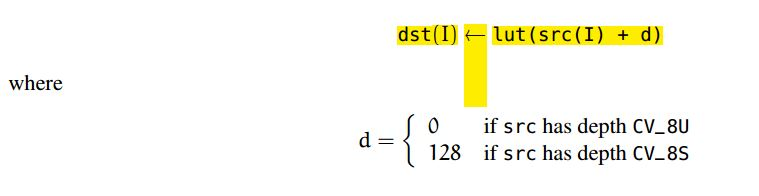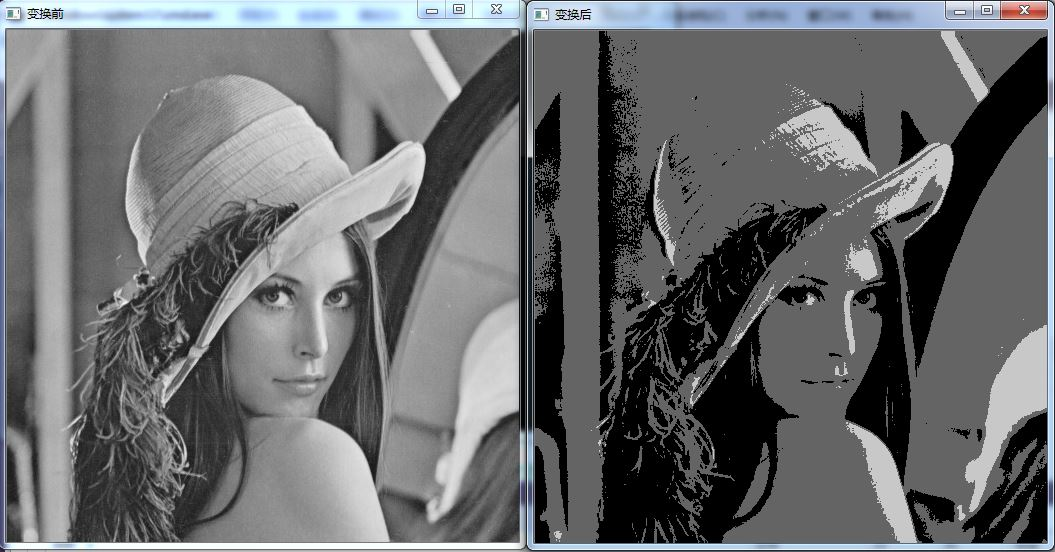# OpenCV（二）如何对图像的像素进行操作int main()
{
string picName="lena.jpg";
uchar table;          //映射表，规定了变换前后灰度值的对应关系 table[gray_value_before]=gray_value_after
for (int i=0;i<256;i++)
{
table[i]=i/100*100;   //这里利用了C++的语言特性i/100只会留下整数部分
}
imshow("变换前",A);
Mat B=ChangeImg (A,table);    //变换函数
imshow ("变换后",B);
waitKey ();
return 0;
}

Mat  ChangeImg(Mat &img,const uchar* table)
{
CV_Assert(img.depth ()!=sizeof(uchar));  //声明只对深度8bit的图像操作
int channels=img.channels ();            //获取图像channel
int nrows=img.rows;                     //矩阵的行数
int ncols=img.cols*channels;             //矩阵的总列数=列数*channel分量数
if (img.isContinuous ())               //判断矩阵是否连续，若连续，我们相当于只需要遍历一个一维数组
{
ncols*=nrows;
nrows=1;                 //一维数组
}
//遍历像素点灰度值
for (int i=0;i<nrows;i++)
{
uchar *p=img.ptr<uchar>(i);    //获取行地址
for (int j=0;j<ncols;j++)
{
p[j]=table[p[j]];           //修改灰度值
}
}
return img;
}


uchar* p = img.data;
for( unsigned int i =0; i < ncol*nrows; ++i)
*p++ = table[*p];

Mat& ScanImageAndReduceIterator(Mat& I, const uchar* const table)
{
// accept only char type matrices
CV_Assert(I.depth() != sizeof(uchar));

const int channels = I.channels();
switch(channels)
{
case 1:
{
MatIterator_<uchar> it, end;
for( it = I.begin<uchar>(), end = I.end<uchar>(); it != end; ++it)
*it = table[*it];
break;
}
case 3:
{
MatIterator_<Vec3b> it, end;
for( it = I.begin<Vec3b>(), end = I.end<Vec3b>(); it != end; ++it)
{
(*it) = table[(*it)];
(*it) = table[(*it)];
(*it) = table[(*it)];
}
}
}

return I;
}

for (int i=0;i<img.rows;i++)
{
const Vec3b* Mpoint=img.ptr <Vec3b>(i);
for (int j=0;j<img.cols;j++)
{
Vec3b intensity=*(Mpoint+j);
cout<<"R:"<<int(intensity)<<" G"<<int(intensity)<<" B"<<int(intensity)<<"    ";
}
cout<<endl;
}int main()
{
string picName="lena.jpg";
Mat lookUpLut(1,256,CV_8UC1);   //建立一个256个元素的映射表
imshow ("变换前",A);
for (int i=0;i<256;i++)
{
lookUpLut.at<uchar>(i)=i/100*100;
}
Mat B;
LUT (A,lookUpLut,B);
imshow ("变换后",B);
waitKey ();
return 0;
}©️2019 CSDN 皮肤主题: 大白 设计师: CSDN官方博客